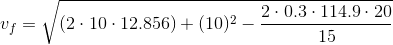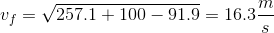# MCAT Physical : Conservation of Energy

## Example Questions

### Example Question #1 : Conservation Of Energy

A ball with mass of 2kg is dropped from the top of a building this is 30m high. What is the approximate velocity of the ball when it is 10m above the ground?

14m/s

20m/s

30m/s

10m/s

25m/s

20m/s

Explanation:

Use conservation of energy. The gravitational potential energy lost as the ball drops from 30m to 10m equals the kinetic energy gained.

Change in gravitational potential energy can be found using the difference in mgh.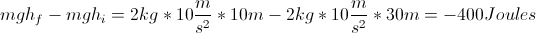So 400 Joules are converted from gravitational potential to kinetic energy, allowing us to solve for the velocity, v.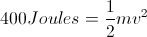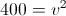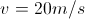### Example Question #2 : Conservation Of Energy

Consider a spring undergoing simple harmonic motion.  When the spring is at its maximum velocity __________.

kinetic energy and potential energy are at a maximum

kinetic energy is at a maximum and potential energy is at a minimum

kinetic energy and potential energy are at a minimum

kinetic energy is at a minimum and potential energy is at a maximum

kinetic energy is at a maximum and potential energy is at a minimum

Explanation:

Kinetic energy is highest when the spring is moving the fastest. Conversely, potential energy is highest when the spring is most compressed, and momentarily stationary. When the force resulting from the compression causes the spring to extend, potential energy decreases as velocity increases.

### Example Question #1 : Conservation Of Energy

A pendulum with a mass of 405kg reaches a maximum height of 2.4m. What is its velocity at the bottommost point in its path?

6.9m/s

48m/s

4.5m/s

17m/s

6.9m/s

Explanation:

First solve for the potential energy of the pendulum at the height of 2.4m.

PE = mgh

PE = (405kg)(10m/s2)(2.4m) = 9720J

This must be equal to the maximum kinetic energy of the object.

KE = ½mv2

9720J = ½mv2

Plug in the mass of the object (405 kg) and solve for v.

9720J = ½(405kg)v2

v = 6.9m/s

### Example Question #4 : Conservation Of Energy

Two children are playing with sleds on a snow-covered hill. Sam weighs 50kg, and his sled weighs 10kg. Sally weighs 40kg, and her sled weighs 12kg. When they arrive, they climb up the hill using boots. Halfway up the 50-meter hill, Sally slips and rolls back down to the bottom. Sam continues climbing, and eventually Sally joins him at the top.

They then decide to sled down the hill, but disagree about who will go first.

Scenario 1:

Sam goes down the hill first, claiming that he will reach a higher velocity. If Sally had gone first, Sam says they could collide.

Scenario 2:

Sally goes down the hill first, claiming that she will experience lower friction and thus reach a higher velocity. If Sam had gone first, Sally says they could collide.

Scenario 3:

Unable to agree, Sam and Sally tether themselves with a rope and go down together.

At the bottom of a neighboring hill, a neighbor watches Sally and Sam come down the hill. Sally is traveling 15m/s and Sam is traveling 10m/s. From the moment the neighbor begins watching, to just after they both come to a stop, who has dissipated more heat in the form of friction? (Assume all friction is lost as heat).

Sam, because he has greater kinetic energy

Sally, because she has less momentum

They dissipate equal amounts because the coeffecients of friction are the same for both

Sally, because she has greater kinetic energy

Sam, because he has more momentum

Sally, because she has greater kinetic energy

Explanation:

Sally has greater kinetic energy in this example than does Sam. From the moment when the neighbor begins watching we can calculate the kinetic energy. Once stopped, all of the kinetic energy will have been dissipated.

Sally's KE = 1/2 (52kg) (15m/s)2 = 5850J

Sam's KE = 1/2 (60kg) (10m/s)2 = 3000J

All of this energy will be dissipated as friction before Sam and Sally come to a stop.

### Example Question #5 : Conservation Of Energy

Ignoring air resistance, which of the following is true regarding the motion of a pendulum?

At the bottom of the oscillation potential energy is at a maximum and kinetic energy is at a minimum

At the bottom of the oscillation potential energy is at a minimum and kinetic energy is at a maximum

At the bottom of the oscillation potential energy is at a minimum and kinetic energy is at a minimum

At the bottom of the oscillation potential energy is at a maximum and kinetic energy is at a maximum

At the bottom of the oscillation potential energy is at a minimum and kinetic energy is at a maximum

Explanation:Energy must be conserved through the motion of a pendulum. Let point 1 represent the bottom of the oscillation and point 2 represent the top. At point 1, there is no potential energy, using point 1 as our "ground/reference," thus all of the system energy is kinetic energy. At point 2, the velocity is zero; thus, the kinetic energy is zero and all of the system energy is potential energy. At the highest point in the swing, potential energy is at a maximum, and at the lowest point in the swing, kinetic energy is at a maximum.

### Example Question #6 : Conservation Of Energy

A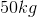boulder drops from rest off of a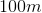cliff. Find its velocity at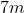before impact.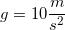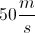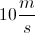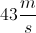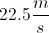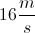Explanation:

Conservation of energy dictates that the initial energy and final energy will be equal.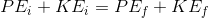In this case, the boulder starts with zero kinetic energy and ends with both kinetic and potential energy.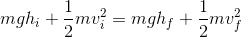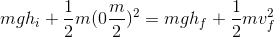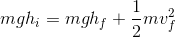We can cancel the mass from each term and plug in the given values to solve for the velocity at a height of.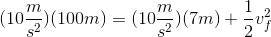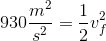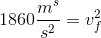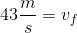### Example Question #2 : Conservation Of Energy

A block of wood is floating in space. A bullet is fired from a gun and hits the block, embedding itself in the wood and generating heat. Which of the following is conserved?

Kinetic energy

Momentum

Mechanical energy

Temperature

Momentum

Explanation:

Momentum is always conserved in a system, when not experiencing external forces.

An inelastic collision can be identified if the two objects stick together after the collision occurs, such as the bullet becoming embedded in the wood. In an inelastic collision, kinetic energy is not conserved. Since mechanical energy is the sum of kinetic and potential energy, mechanical energy is also not conserved. This lack of conservation is due to the conversion of some of the kinetic energy to heat and sound. The kinetic energy decreases as the heat energy increases, resulting in a non-constant temperature.

### Example Question #8 : Conservation Of Energy

A rock is dropped from a given height and allowed to hit the ground. The velocity of the rock is measured upon impact with the ground. Assume there is no air resistance.

In order for the velocity of the rock to be doubled before impact, which of the following is necessary?

Halve the height from which the rock is dropped

Reduce by 25% the height from which the rock is dropped

Reduce by 75% the height from which the rock is dropped

Quadruple the height from which the rock is dropped

Double the height from which the rock is dropped

Quadruple the height from which the rock is dropped

Explanation:

We can compare height and velocity by comparing the equations for potential and kinetic energy. This is possible because the rock initially has no kinetic energy (velocity is zero) and has no potential energy upon impact (height is zero). Using conservation of energy will yield the comparison below.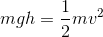Because velocity is squared in the equation for kinetic energy, it requires a quadrupling of height in order to double the velocity.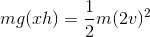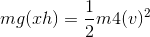### Example Question #1 : Conservation Of Energy

A stone of mass m sits atop a hill of height h.  As it rolls downhill, which of the following is true?

1. Half way down the hill,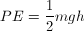2. Half way down the hill,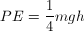3. Half way down the hill,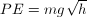4. Half way down the hill, PE still equals mgh.
5. None of these is true.

1

5

2

3

4

1

Explanation:

4. Choice 1 is correct because initially, all of the mechanical energy in the stone was potential energy and none was kinetic energy:  ME = KE + PE.  PE is “stored” in the stone-hill system by rolling the stone up hill.  It is obvious that it takes half as much energy to roll the stone half way up the hill, compared with rolling it to the top.  At the bottom of the hill, all of the PE will have been converted into KE, given by the formula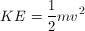Since the PE was ½ mgh when rolling the stone half way up hill, it is the same as it rolls down hill.

### Example Question #10 : Conservation Of Energy

An empty mining cart has a mass of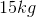and is traveling down a track that has a slope of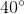to the horizontal. The cart is traveling at a rate of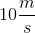when an operator notices a disturbance on the track ahead and locks the wheels of the cart. What is the speed of the cart after it has traveled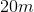with the wheels locked?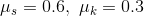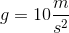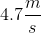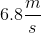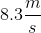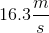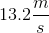Explanation:

We need the equation for conservation of energy for this problem: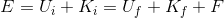We can eliminate final potential energy if we set the final height to be zer. We are solving for final velocity, so let's rearrange for final kinetic energy.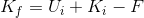Substituting our equations for each variable, we get: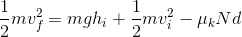Rearranging for final velocity we get: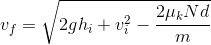If you derive this formula and are unsure of your work, simply check your units. Each term under the square root has units of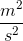, which will ultimately give us units of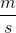, which is what we want.

We have values for all variables except two: height and normal force.

Let's calculate the height. We know that the between the initial and final states, the cart has traveled 20 meters down a slope of 40 degrees. Therefore, we can calculate height with the formula: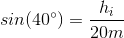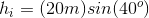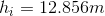Now we just need to find the normal force. The following diagram will help visualize this calculation.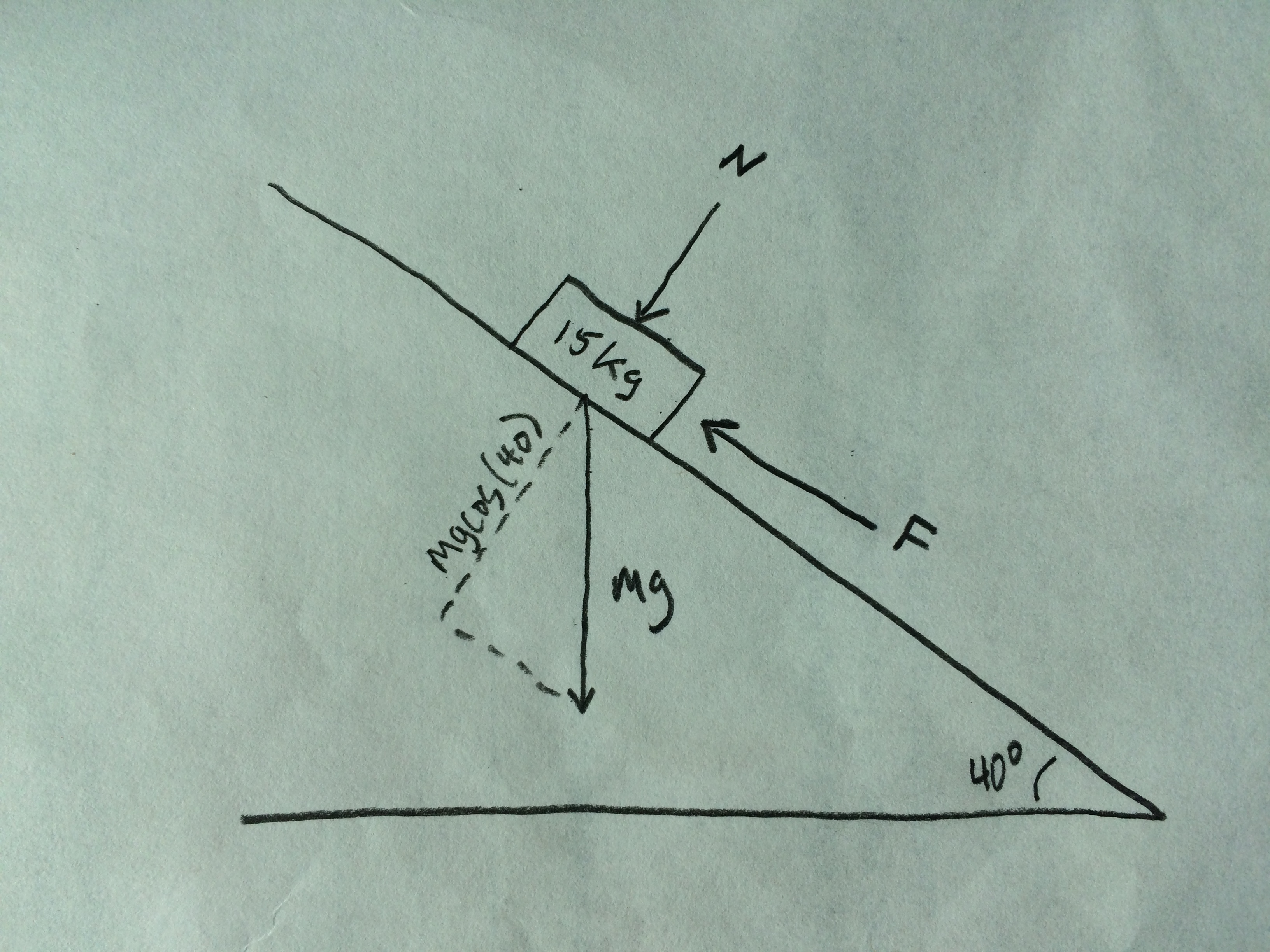If you are unsure whether to use sine or cosine, think about it practically. As the angle gets less and less, the normal force is going to get larger. This is characteristic of a cosine function.

Therefore, we can say that: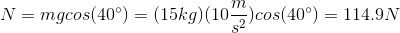Now that we have all of our variables, it's time to plug and chug: